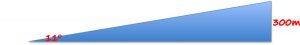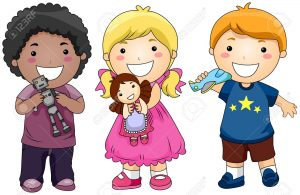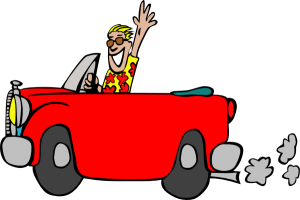## November 2018 Problem of the Month Solution

Question: An airplane climbs at an angle of 11 degrees with the ground. What is the distance the airplane has travelled when it has attained an altitude of 300 metres?

Solution:

First, draw a right triangle.  Label the angle of inclination of the plane and the altitude.The question is asking for the diagonal distance, which corresponds to the distance that the plane has travelled.  Therefore, we are finding the hypotenuse of the triangle.

By using SOH CAH TOA, we should see that we are using the sine ratio, as follows:$\sin { \quad \theta } =\quad \frac { opposite }{ hypotenuse }$$\sin{{ \quad 11 }^{ o } } =\quad \frac { 300 }{ d }$$d\quad *\quad \sin{ \quad { 11 }^{ o } } =\quad 300$$d\quad =\quad \frac { 300 }{ \sin{ \quad { 11 }^{ o } } } \quad$$d\quad =\quad 1572.25m$

Therefore, the plane has flown approximately 1572 metres when it has reached an altitude of 300 metres.

## November Problem of the Month

Question: An airplane climbs at an angle of 11 degrees with the ground.  What is the distance the airplane has travelled when it has attained an altitude of 300 metres?## May Problem of the Month Solution

Question: Four children have small toys. The first child has 1/10 of the toys, the second child has 12 more toys than the first, the third child has one more toy of what the first child has and the fourth child has double the third child. How many toys are there?

Solution:

1. x : the total number of toys
2. x/10 : the number of toys for first child
3. x/10 + 12 : the number of toys for second child
4. x/10 + 1 : the number of toys for the third child
5. 2(x/10 + 1) : the number of toys for the fourth child
6. x/10 + x/10 + 12 + x/10 + 1 + 2(x/10 + 1) = x
7. x = 30 toys : solve for x

## May Problem of the Month

Question: Four children have small toys. The first child has 1/10 of the toys, the second child has 12 more toys than the first, the third child has one more toy of what the first child has and the fourth child has double the third child. How many toys are there?## February Problem of the Month SolutionQuestion: A mother is three times as old as her daughter. Six years ago, the mother’s age was six times that of her daughter. How old are they now?

Solution:

Let x represent the daughter’s age.
Therefore, 3x is the mother’s age.
6(x – 6) = 3x – 6
6x – 36 = 3x – 6 –> 6x – 3x = -6 + 36
3x = 30
x = 10
Therefore, the daughter’s is 10 years old and the mother is 30 years old.

## February Problem of the MonthQuestion: A mother is three times as old as her daughter. Six years ago, the mother’s age was six times that of her daughter. How old are they now?

## November Problem of the Month

Four children have small toys. The first child has 1/10 of the toys, the second child has 12 more toys than the first, the third child has one more toy of what the first child has and the fourth child has double the third child. How many toys are there?

## September 2017 Problem of the Month Solution

Question: At 11:00 a.m., John started driving along a highway at constant speed of 50 miles per hour. A quarter of an hour later, Jimmy started driving along the same highway in the same direction as John at the constant speed of 65 miles per hour. At what time will Jimmy catch up with John?

Solution:

Let t be the number of hours, from 11:00 am, when Jimmy catches up with John. The time that Jimmy will have to drive to catch up with John is t – 1/4: he starts a quarter of an hour late. When Jimmy catches up with John, they would have traveled the same distance. Hence

50 t = 65 (t – 1/4)

Solve for t

50t = 65t – 65/4

t = 65/60 = 1.083 hours = 1hour and 5 minutes

Jim will catch up with John at

11:00 am + 1 hour , 5 minutes = 12:05 pm

## September 2017 Problem of the MonthAt 11:00 a.m., John started driving along a highway at constant speed of 50 miles per hour. A quarter of an hour later, Jimmy started driving along the same highway in the same direction as John at the constant speed of 65 miles per hour. At what time will Jimmy catch up with John?

## August 2017 Problem of the Month Solution

Question: The cost of tickets for a play is $3.00 for adults and$2.00 for children. 350 tickets were sold and $950 was collected. How many tickets of each type were sold? Step 1: Set up a table with quantity and value.  quantity value total$3 tickets $2 tickets together Step 2: Fill in the table with information from the question. The cost of tickets for a play is$3.00 for adults and $2.00 for children. 350 tickets were sold and$950 was collected. How many tickets of each type were sold?

 Let x = number of $3 tickets y = number of$2 tickets Total = quantity × value

 quantity value total $3 tickets x 3 3x$2 tickets y 2 2y together 350 950

Step 3: Add down each column to get the equations

x + y = 350                    (equation 1)
3x + 2y = 950                 (equation 2)

Use Substitution Method
Isolate variable x in equation 1

x = 350 – y                     (equation 3)

Substitute equation 3 into equation 2

3(350 – y) + 2y = 950
1050 – 3y + 2y = 950
3y – 2y = 1050 – 950
y = 100

Substitute y = 100 into equation 1

x + 100 = 350
x = 250

Answer: 250 $3 tickets and 100$2 tickets were sold.